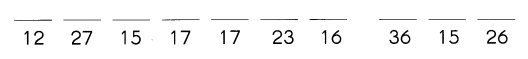# Math in Focus Grade 3 Chapter 8 Practice 5 Answer Key Division with Regrouping in Tens and Ones

Practice the problems of Math in Focus Grade 3 Workbook Answer Key Chapter 8 Practice 5 Division with Regrouping in Tens and Ones to score better marks in the exam.

## Math in Focus Grade 3 Chapter 8 Practice 5 Answer Key Division with Regrouping in Tens and Ones

Question 1.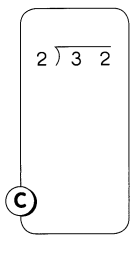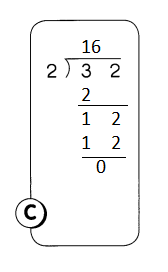Explanation:
With the help of base ten blocks regrouping is done
3 tens and 2 ones is converted to 2 tens and 12 ones

Question 2.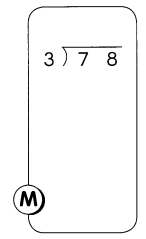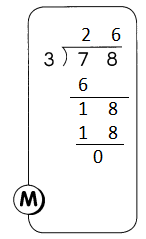Explanation:
With the help of base ten blocks regrouping is done
7 tens and 8 ones is converted to 6 tens and 18 ones

Question 3.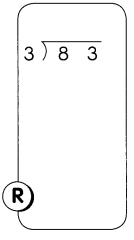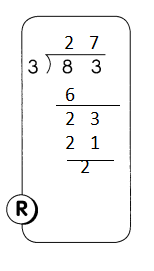Explanation:
With the help of base ten blocks regrouping is done
8 tens and 3 ones is converted to 6 tens and 23 ones

Question 4.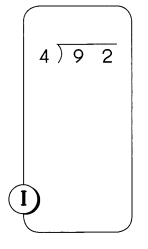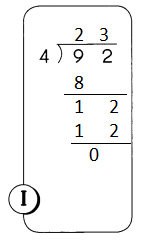Explanation:
With the help of base ten blocks regrouping is done
9 tens and 2 ones is converted to 8 tens and 12 ones

Question 5.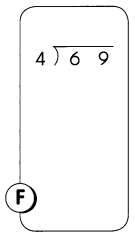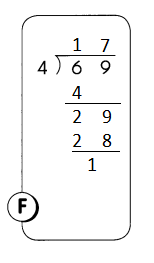Explanation:
With the help of base ten blocks regrouping is done
6 tens and 9 ones is converted to 4 tens and 29 ones

Question 6.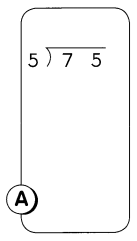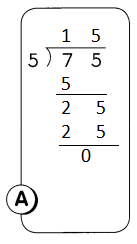Explanation:
With the help of base ten blocks regrouping is done
7 tens and 5 ones is converted to 5 tens and 25 ones

Question 7.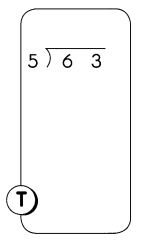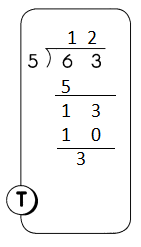Explanation:
With the help of base ten blocks regrouping is done
6 tens and 3 ones is converted to 5 tens and 13 ones

Question 8.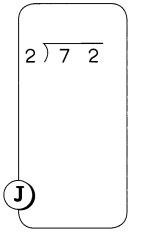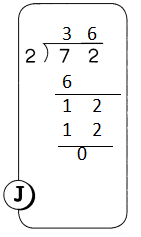Explanation:
With the help of base ten blocks regrouping is done
7 tens and 2 ones is converted to 6 tens and 12 ones

What kind of jam cannot be eaten?

Match the letters to the quotients below to find out.

Question 9.